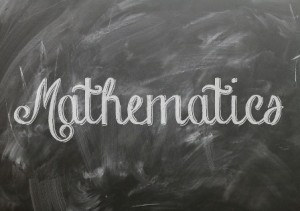# Indeterminate forms in MathematicsAn indeterminate form in mathematics is an algebraic expression whose value cannot be determined definitely. There are 7 known indeterminate forms, which are : 00, 0/0, 1∞, ∞ − ∞, ∞/∞, 0 × ∞, and ∞0.

When trying to evaluate an algebraic expression or function, we use limits if it is not possible to determine the exact value. That is, we try to find the limiting value of the function at some point. Yet, at times we arrive at certain forms of limits for which, merely finding the limiting behaviour of individual parts is not sufficient to actually determine the overall limit. These forms are said to be indeterminate. For example, the limit of the function f(x)/g(x) would give rise to the form 0/0 if the individual limits of each function f(x) and g(x) are zero for some limiting value of x. This is an indeterminate form since the value of the overall limit actually depends on the limiting behavior of the combination of the two functions, and not on the individual functions.

Many mathematicians have attempted to arrive at feasible methods to find or solve these indeterminate forms. French mathematician Guillaume de L’Hôpital is probably the most successful of all, introducing the L’Hopital’s rule (which is also known as Bernoulli’s rule since Johann Bernoulli also played a part in the solution) to arrive at a limiting value for the two indeterminate forms 0/0 and ∞/∞.

The L’Hopital’s rule says that if f(x) and g(x) are two functions whose individual limits as x tends to some value c, are either both 0 or both ∞, then: lim xc [f(x)/g(x)] = lim xc [f ’(x)/g ’(x)], provided that the limit exists. In other words, the limit of the division between two functions that both tend to either 0 or ∞, is equal to the same limit of the division between the first derivatives of the two functions; as long is there exists such a limit. This has proven very useful in many mathematical problems, and is widely used in calculus.

If you are studying calculus for WACE exams at higher levels, you will come across limits and indeterminate forms, and will get a chance to apply L’Hopital’s rule. If you have questions about indeterminate forms, you could try to obtain WACE help and ask any online tutor in Australia about it. Most math tutors in Australia would be able to help you with problems related to indeterminate forms.Article Source: article biz